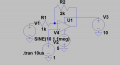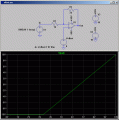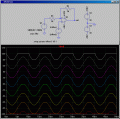# Inverting amplifer question

#### Rock Slate

Joined Jul 22, 2015
27Hi guys,

I have a question. The diagram below shows the inverting amplifier. The sine wave has a DC offset of about 10V. However the source connected to the non inverting terminal is a DC source which has a value of 1V. In this case does the concept of virtual ground still apply. As far as I can tell , all the current still flows through resistors R1, R2 and none into the input of the opamp. Can anyone tell me why even if I replace the non inverting terminal source with ground, the potential at the non inverting terminal is not close to zero?#### Attachments

• 984 bytes Views: 4

#### ErnieM

Joined Apr 24, 2011
8,171
"Virtual ground" is an approximation for a particular curcuit. Make another circuit and you need a different approximation.

Here's something closer to reality: Given an inverting op amp circuit IF the output is not clipped then the two input terminals will be so very close in value you may assume they are the same.

For the inverter it follows that since the + input is grounded you may also assume the - input is grounded. BUT... move the + input a volt above ground and guess what? The - input goes the same amount providing the output is not clipped.

Now, what and where is that 10V offset on what sine wave?

Oh, and please jam together the parts on your schematic lots more. I can still almost read it as is.#### Rock Slate

Joined Jul 22, 2015
27
I have attached the LTSPICE file. I dont think the opamp is clipped. You can take a look at it. However there still exists a large potential difference between the 2 terminals.

#### Bordodynov

Joined May 20, 2015
2,890
Your amplifier can not work properly. The output level is almost equal to -5V. see the amplitude of the output signal, and you'll understand. It is necessary to insert a capacitor. Especially for you: a real gain of the amplifier at high operating frequency of the incident. The value of the feedback factor will also be small. Then, the distortion is great.

#### Attachments

• 1.2 KB Views: 5
•Rock Slate

#### Rock Slate

Joined Jul 22, 2015
27
Ah okay. So for an opamp to work properly we need to remove the DC input to maintain the gain. Is that the way of looking at it

#### MikeML

Joined Oct 2, 2009
5,444
The key to understanding what is going on is this simple simulation. Note what V(out) does as a function of V(offset). The ground is not "virtual"; the ground is 0V. You can bias the opamp into a region where it can "amplify" the 1Vpp input riding on a 10V level only if V(offset) puts the amplifier into a regime where its inputs are within the allowed common-mode range.Note the use of a .DC simulation using a voltage sweep to show what is going on. The x-axis in the plot is V(offset). The y-axis shows V(out) as V(offset) sweeps from oV to 10V. Since the gain of the amplifer is 2, then V(offset) would have to be between ~5.3V and 8V to accomodate the 2Vpp sine output without clipping caused by running into the power supply rails.

Note the use of better drafting practices to make a more readable LTSpice schematic.

#### Rock Slate

Joined Jul 22, 2015
27
Just one question. On the y axis of the Dc analysis , why do you say that the V(offset) has to be between 4 and -4V. I understand the gain is 2 , so the response should be between -4V and 4V. But I am still unsure as to how you got 5.3-8V. For example what would happen if I supply 5.2. Would the portion just above -4V get clipped? Or is it something else?

#### MikeML

Joined Oct 2, 2009
5,444
Just one question. On the y axis of the Dc analysis , why do you say that the V(offset) has to be between 4 and -4V. I understand the gain is 2 , so the response should be between -4V and 4V. But I am still unsure as to how you got 5.3-8V. For example what would happen if I supply 5.2. Would the portion just above -4V get clipped? Or is it something else?
I didn't say -4V to 4V!
In post #6 I said that for the sine wave to be unclipped, V(offset) should be between ~5.3V and 8V. Let's use LTSpice to ask that question in more detail. Here I repeat the .TRAN sim at various values of V(offset) ranging from 3V (Red) to 10V (White) in steps of 1V. V(out) is still clipped at V(offset)=4V, but unclipped at V(offset)=5V, so my guess was a bit off.

Unclipped at V(offset)=9V (Gray) , but clipped at V(offset)=10V (White), so a bit more than my guess of 8V. Note that you had the sine wave at 1MHz, and that (universal) opamp is slew-rate limited at that frequency. I reduced the input to 100kHz, and the wave looks more sinosoidal...Note that it is easy to find V(offset) that centers the sinosoid on 0V. The left end of R1 is centered at 10V. We want the right end of R2 to be at 0V. The opamp forces the voltage the inverting input to be equal to Voffset, so I = E/R = (10-Voffset)/R1 = (Voffset-0)/R2.
R2(10-Voffset) = R1Voffset
20000-2000Voffset = 1000Voffset
3000Voffset= 20000
Voffset = 20000/3000 = 6.667

Look familiar?

Another way of thinking about this is that if it were not for the rails on the opamp, 10V input with a gain of -(R2/R1) = -2 would make -20V. We want to add in Voffset to move the output from -20V to 0V (net +20V). The gain relative to the non-inverting input where Voffset is introduced is 1+R2/R1 = 1+2 = 3, so 20/3 = 6.667V,

so -20V + 6.667*3= 0V.

Last edited:
•Rock Slate